Courses

# Short Answer Type Questions- Surface Areas and Volumes Class 10 Notes | EduRev

## Class 10 : Short Answer Type Questions- Surface Areas and Volumes Class 10 Notes | EduRev

The document Short Answer Type Questions- Surface Areas and Volumes Class 10 Notes | EduRev is a part of the Class 10 Course Class 10 Mathematics by VP Classes.
All you need of Class 10 at this link: Class 10

Q1. A metallic sphere of radius 4.2 cm is melted and recast into the shape of a cylinder of radius 6 cm.

Find the height of the cylinder.

Sol. Here, radius (r) = 4.2 cm

∴ Volume of the sphere  = 4/3 πr3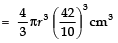Let the height of the cylinder  =  h
∵ Radius of the cylinder (R)  =  6 cm
∴ Volume of the cylinder = πR2h =  π × (6)2 × h

Since,[Volume of the cylinder] = [Volume of the sphere]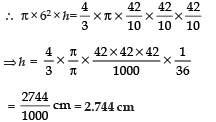Q2. The figure shows a decorative block which is made of two solids—a cube and a hemisphere. The base of the block is a cube with edge 5 cm and the hemisphere, fixed on the top, has a diameter of 4.2 cm.

Find the total surface area of the block.   [Take π = 22/7]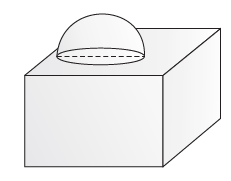Sol. ∵ Total surface area of a cube = = 6 × (side)2
∴ Total surface area of the cubical block
= 6 × (5)2 − [Base area of the hemisphere]
= 150 − πr2
Now, total surface area of the solid

= 150 − πr2 + 2πr2 = 150 + πr2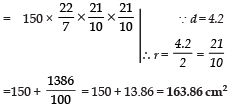Q3. A toy is in the form of a cone mounted on a hemisphere with same radius. The diameter of the base of the conical portion is 7 cm and the total height of the toy is 14.5 cm. Find the volume of the toy.

[Use π = 22/7]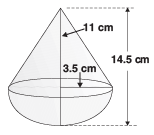Sol.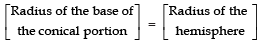⇒ r = 3.5 cm,

Height of the conical portion (h) = 14.5 − 3.5 = 11 cm

Now, Volume of the toy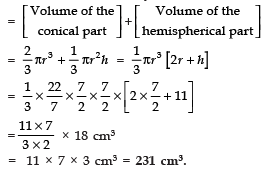Q4. In the figure, the shape of a solid copper piece (made of two pieces) with dimensions as shown. The face ABCDEFA has uniform cross section. Assume that the angles at A, B, C, D, E and F are right angles. Calculate the volume of the piece.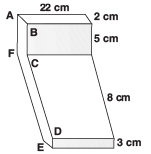Sol. Since, volume of a cuboid = lbh
∴ Volume of horizontal piece
= 22 × (8 + 2) × 3 cm3 = 22 × 10 × 3 cm3
=  660 cm3 Volume of the vertical piece
= 22 × 2 × 5 cm3 = 220 cm3
∴Total volume of the piece
= 660 cm3 + 220 cm3 = 880 cm3

Q5. A toy is in the form of a cone mounted on a hemisphere of common base radius 7 cm. The total height of the toy is 31 cm. Find the total surface area of the toy. [Use π = 22/7]

Sol. Height of the cone, h = 31 − 7 = 24 cm

r = 7 cm.

Now, Slant height, l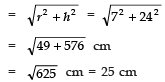∴ Total surface area of the toy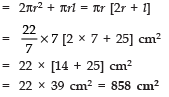Q6. A sphere of diameter 6cm is dropped in a right circular cylindrical vessel partly filled with water. The diameter of the cylindrical vessel is 12 cm. If the sphere is completely submerged in water, by how much will the level of water rise in the cylindrical vessel?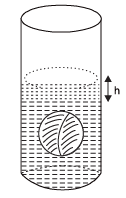Sol. Let the water level raised in cylindrical vessel be 'h' cm
Since, the water raised in the form of a cylinder
∵ Volume of the raised water = π (6)2 h Now,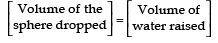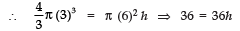or h = 1 cm

Thus, the water level is raised by 1 cm

Offer running on EduRev: Apply code STAYHOME200 to get INR 200 off on our premium plan EduRev Infinity!

132 docs

,

,

,

,

,

,

,

,

,

,

,

,

,

,

,

,

,

,

,

,

,

;